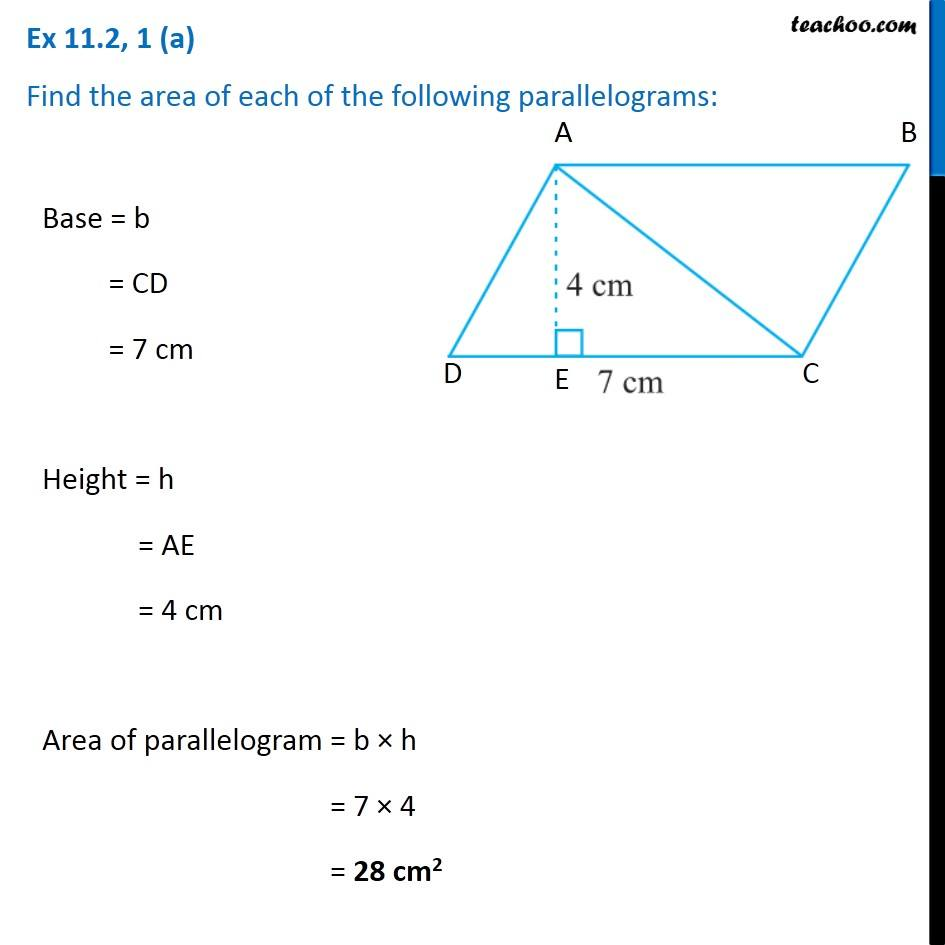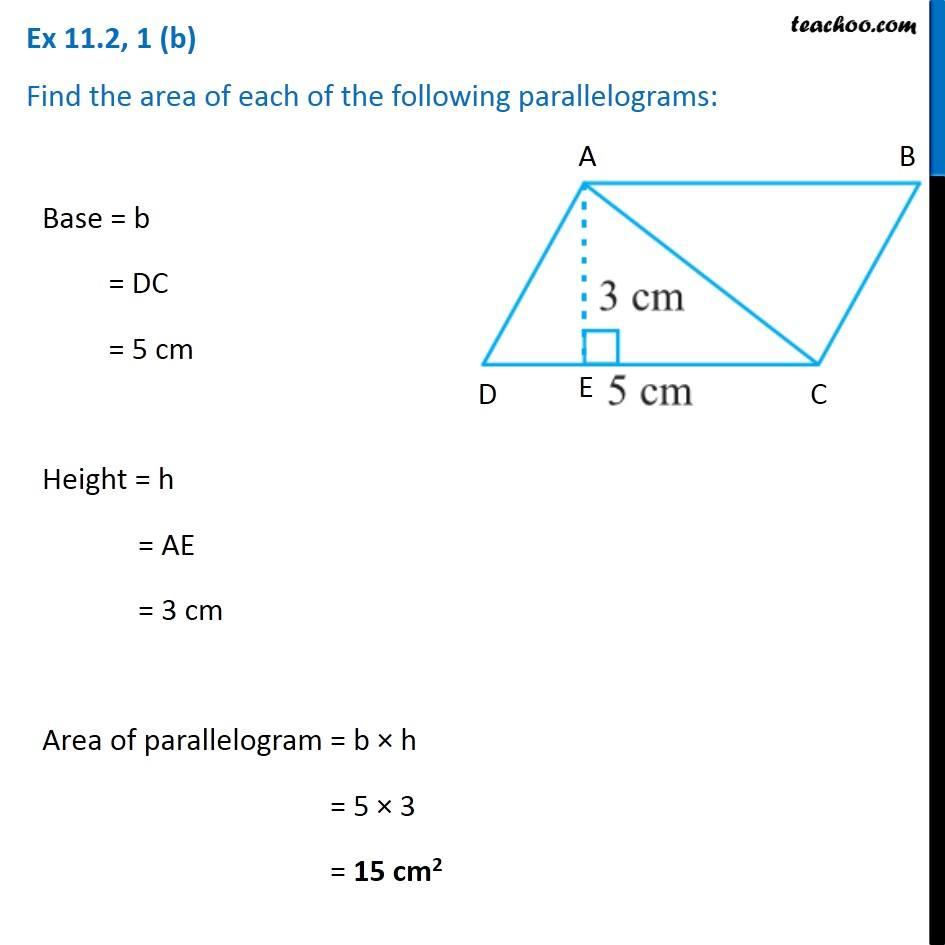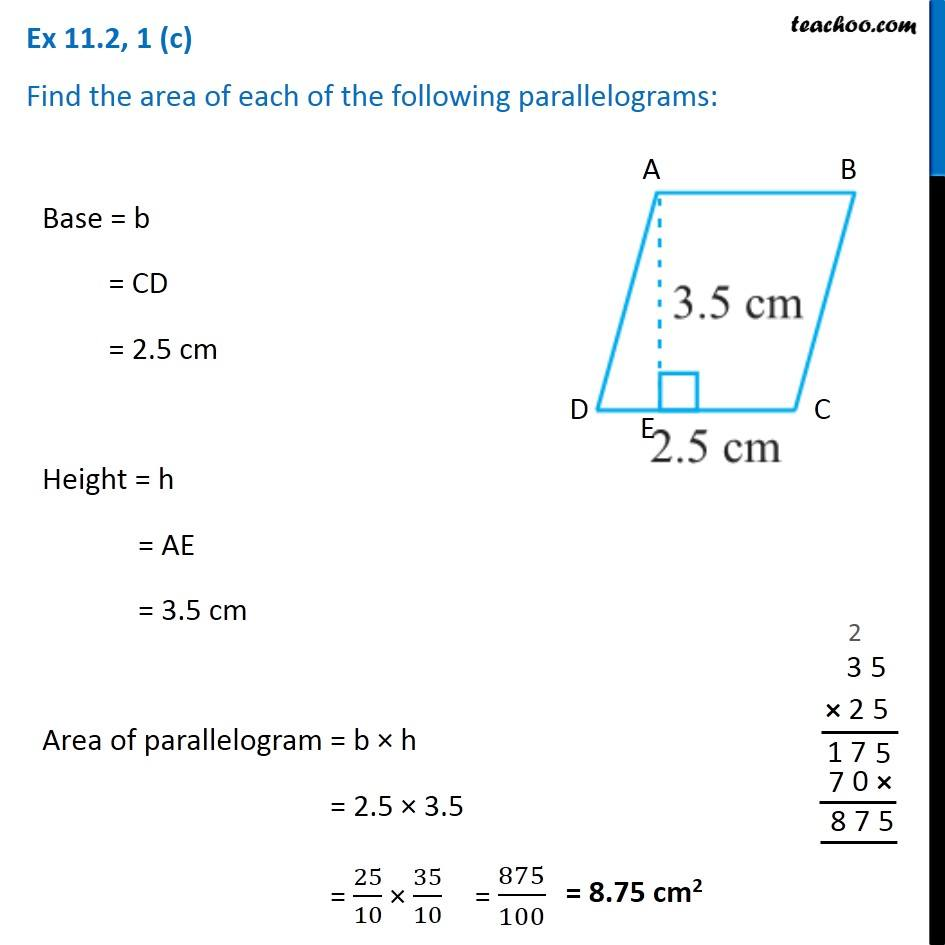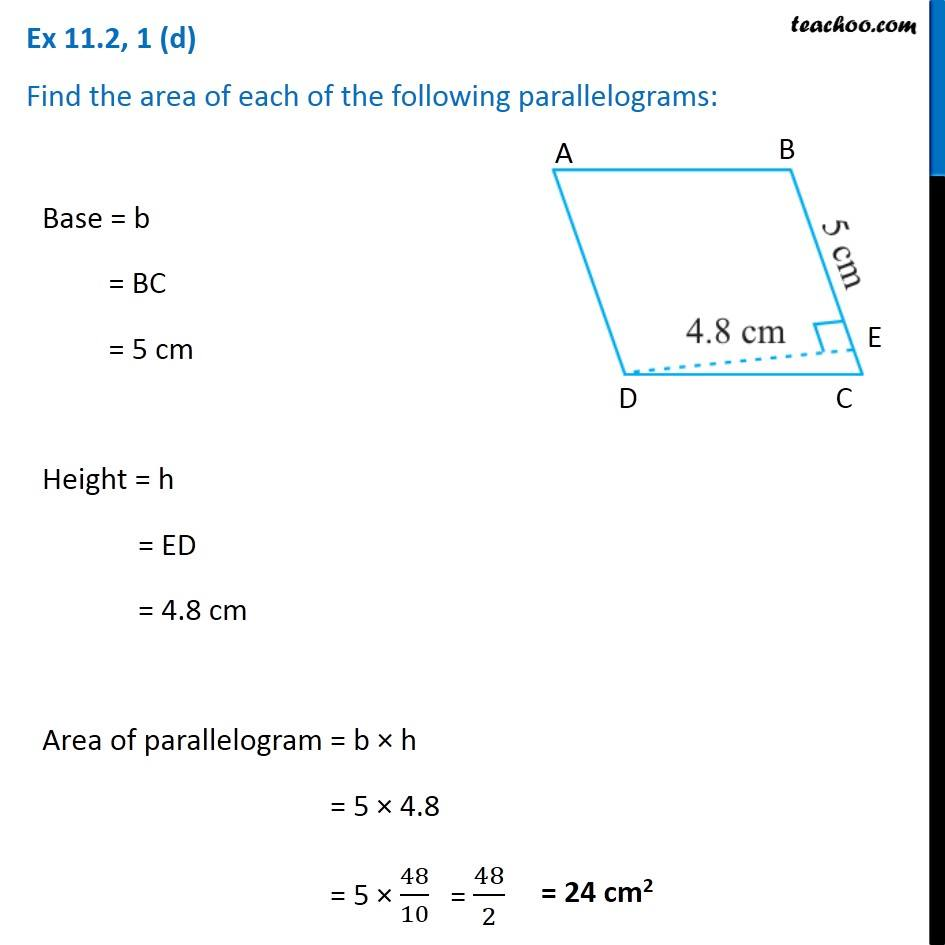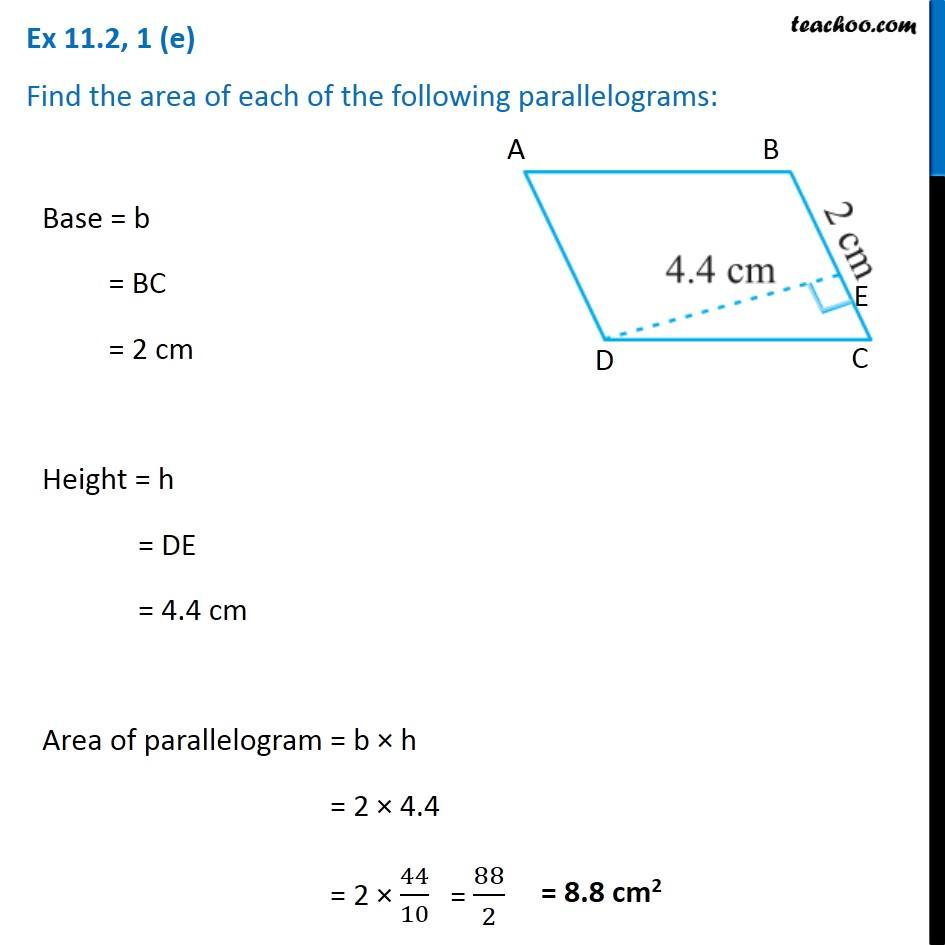Subscribe to our Youtube Channel - https://you.tube/teachoo

1. Chapter 11 Class 7 Perimeter and Area
2. Serial order wise
3. Ex 11.2

Transcript

Ex 11.2, 1 (a) Find the area of each of the following parallelograms: Base = b = CD = 7 cm Height = h = AE = 4 cm Area of parallelogram = b × h = 7 × 4 = 28 cm2 Ex 11.2, 1 (b) Find the area of each of the following parallelograms: Base = b = DC = 5 cm Height = h = AE = 3 cm Area of parallelogram = b × h = 5 × 3 = 15 cm2 Ex 11.2, 1 (c) Find the area of each of the following parallelograms: Base = b = CD = 2.5 cm Height = h = AE = 3.5 cm Area of parallelogram = b × h = 2.5 × 3.5 = 25/10 × 35/10 Ex 11.2, 1 (d) Find the area of each of the following parallelograms: Base = b = BC = 5 cm Height = h = ED = 4.8 cm Area of parallelogram = b × h = 5 × 4.8 = 5 × 48/10 = 48/2 Ex 11.2, 1 (e) Find the area of each of the following parallelograms: Base = b = BC = 2 cm Height = h = DE = 4.4 cm Area of parallelogram = b × h = 2 × 4.4 = 2 × 44/10 = 88/2 = 8.8 cm2

Ex 11.2